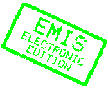MATHEMATICA BOHEMICA, Vol. 130, No. 2, pp. 185-202 (2005)

# Realizable triples for stratified domination in graphs

## Ralucca Gera, Ping Zhang

Ralucca Gera, Ping Zhang, Department of Mathematics, Western Michigan University, Kalamazoo, MI 49008, USA, e-mail: ping.zhang@wmich.eduAbstract: A graph is $2$-stratified if its vertex set is partitioned into two classes, where the vertices in one class are colored red and those in the other class are colored blue. Let $F$ be a $2$-stratified graph rooted at some blue vertex $v$. An $F$-coloring of a graph $G$ is a red-blue coloring of the vertices of $G$ in which every blue vertex $v$ belongs to a copy of $F$ rooted at $v$. The $F$-domination number $\gamma_F(G)$ is the minimum number of red vertices in an $F$-coloring of $G$. In this paper, we study $F$-domination where $F$ is a red-blue-blue path of order 3 rooted at a blue end-vertex. It is shown that a triple $(\cA, \cB, \cC)$ of positive integers with $\cA\le\cB\le2 \cA$ and $\cB\ge2$ is realizable as the domination number, open domination number, and $F$-domination number, respectively, for some connected graph if and only if $(\cA, \cB, \cC) \ne(k, k, \cC)$ for any integers $k$ and $\cC$ with $\cC> k \ge2$.

Keywords: stratified graph, $F$-domination, domination, open domination

Classification (MSC2000): 05C15, 05C69

Full text of the article:

[Previous Article] [Next Article] [Contents of this Number] [Journals Homepage]
© 2005–2010 FIZ Karlsruhe / Zentralblatt MATH for the EMIS Electronic Edition